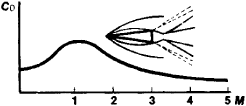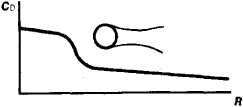# aerodynamic coefficients

The following article is from The Great Soviet Encyclopedia (1979). It might be outdated or ideologically biased.

## Aerodynamic Coefficients

dimensionless quantities which characterize the aerodynamic force and moment acting on an object moving through a fluid or gaseous medium. The aerodynamic coefficients of a force CR are defined as the ratio of the aerodynamic force R to the velocity head (ρ v2)/2 and the characteristic area 5, and the aerodynamic coefficient of the moment CM, as the ratio of the aerodynamic moment M to (ρ v2)/2, S, and the characteristic length l —that is,

CR = R/½ρ v2S CM = M/½ ρ v2Sl

where ρ is the density of the medium through which the object is moving and ν is the velocity of the object relative to this medium. The characteristic dimensions are chosen rather arbitrarily. For an airplane, for example, S is the surface area of the supporting wings (in a plane) and / is the length of the wing chord; for a rocket, S is the surface area of the maximum cross section and / is the length of the rocket. If the aerodynamic force and moment are resolved into components along the coordinate axes, the following are obtained: the aerodynamic drag coefficient (C0), the lift and cross forces coefficients (Cu and Cz respectively), and also the aerodynamic coefficients of the rolling, yawing, and pitching moments.

The expression of aerodynamic forces and moments in the form of aerodynamic coefficients is very important in aerodynamic studies and calculations, simplifying them considerably. Thus, for example, the aerodynamic force acting on an airplane can attain values in the hundreds and thousands of kilonewtons (kN; tens and hundreds of ton-forces); the same force acting on the model of this plane which is being tested in a wind tunnel will be tens of newtons, but the aerodynamic coefficients for the airplane and the model will be equal.Figure 1. Dependence of the aerodynamic drag coefficient of a cone on the Mach numberFigure 2. Dependence of the aerodynamic drag coefficient of a sphere on the Reynolds (R) number

For large devices flying at low altitude with subsonic velocity for which the Mach number is less than 0.2, the aerodynamic coefficient depends mainly on the shape of the aircraft and the angle of attack (the angle between the characteristic plane and the direction of velocity of the airplane). Generally, the aerodynamic coefficients depend on the viscosity and compressibility of the gas, which is characterized by dimensionless similarity criteria—the Mach number and the Reynolds number (see Figures 1 and 2).

M. IA. IUDELOVICH

## aerodynamic coefficients

Nondimensional coefficients for aerodynamic forces and moments. The most common are the coefficients of lift and drag (i.e., CL and CD).